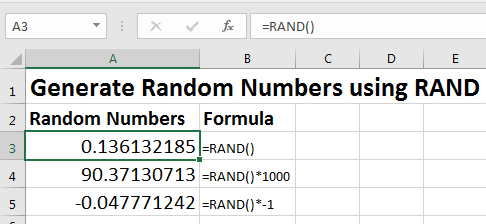# How to Use RAND Function in Excel

The RAND function returns a random number between 1 and 0. The returned random number can have up to 15 decimal digits.Each time a worksheet is calculated RAND function updates a new number. To fix the random number you got, copy and paste it as value.

RAND function Syntax

=RAND()

RAND function doesn't need any argument.

#### How to use RAND functions?

To get a random number between 1 and 0 just write RAND in any cell in excel.
To get random number in multiple cells at once, just select multiple cells and write RAND formula in formula bar and hit CTRL+ENTER key combination on keyboard. And walla! Here you have your random numbers in selected cell.

Now if you want whole random numbers between a specified range, you should use RANDBETWEEN function.

Some random related article:

Get Random numbers between two numbers

Generate Random Phone Numbers

Excel Random Selection

So yeah, this how you use RAND function in excel to generate random numbers between 1 and 0.

Popular Articles:

50 Excel Shortcuts to Increase Your Productivity

How to use the VLOOKUP Function in Excel

How to use the COUNTIF function in Excel 2016

How to Use SUMIF Function in Excel

Terms and Conditions of use

The applications/code on this site are distributed as is and without warranties or liability. In no event shall the owner of the copyrights, or the authors of the applications/code be liable for any loss of profit, any problems or any damage resulting from the use or evaluation of the applications/code.